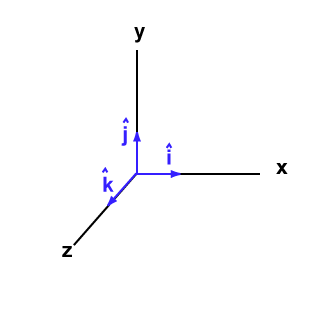0

# More PropertiesAnother property is:

u × (v + w) = u × v + u × w .

To see this, plug into the definition of the cross product.

Often the unit vectors in the direction of the x, y, and z axes (in whatever coordinate frame is currently being used) are called i, j, and k. Sometimes (as in the picture), little hats are put on each letter.

Since the length of each vector is one, and they are mutually perpendicular (so the sine of the angle between any two is 1.0), the following is true:

 ``` i × j = k j × k = i k × i = j ``` ``` i × k = -j k × j = -i j × i = -k ```

Rather than memorize this, just draw the diagram and use the right hand rule.

### QUESTION 10:

What is each of the following:

```i × i   =

j × j   =

k × k   =
```